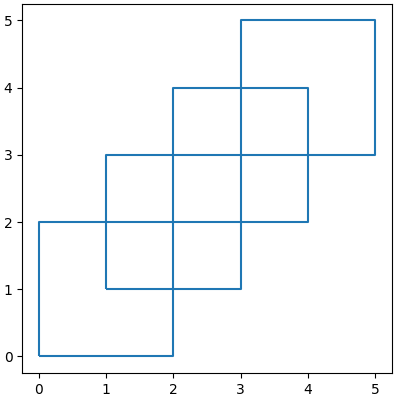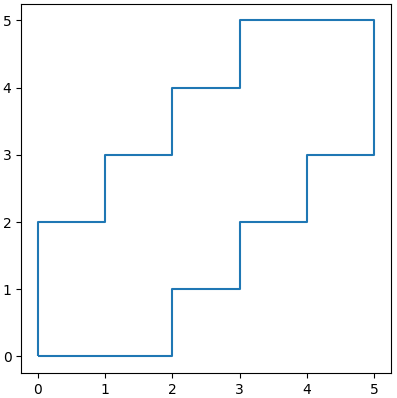Let’s first create some polygons to merge.

from matplotlib import pyplot as plt
import geopandas as gpd
from shapely.geometry import Polygon

poly1 = Polygon([(0,0), (2,0), (2,2), (0,2)])
poly2 = Polygon([(2,2), (4,2), (4,4), (2,4)])
poly3 = Polygon([(1,1), (3,1), (3,3), (1,3)])
poly4 = Polygon([(3,3), (5,3), (5,5), (3,5)])
polys = [poly1, poly2, poly3, poly4]

gpd.GeoSeries(polys).boundary.plot()
plt.show()Now, let’s call the unary_union() function in shapely.ops, which we can directly apply to a list of polygons, and plot the result.

from shapely.ops import unary_union

mergedPolys = unary_union(polys)

gpd.GeoSeries([mergedPolys]).boundary.plot()
plt.show()